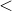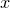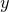## 11.52.19 cnrange

```set c<n>range [ <range> ]
[ reversed | noreversed ]
[ renormalise | norenormalise ]
```

The set c<n>range command changes the range of ordinate values represented by different colors in the colormap plot style, and in the case of the set c1range command, also by contours in the contourmap plot style. The value <n> should be an integer in the range 1–4.

### Contour Maps

The effect of the set c1range command upon the set of ordinate values for which contours are drawn in the contourmap plot style is dependent upon whether the set contours command has been supplied with a number of contours to draw, or a list of explicit ordinate values for which they should be drawn. In the latter case, the set c1range command has no effect. In the former case, the contours are evenly spaced, either linearly or logarithmically depending upon the state of the logscale c1 setting, between the minimum and maximum ordinate values supplied to the set c1range command. If an asterisk (*) is supplied in place of either the minimum and/or the maximum, then the range of values used is automatically scaled to fit the range of the data supplied.

### Color Maps

The color of each pixel in a color map is determined by the colormap setting. This should contain an expression that evaluates to a color object, e.g. rgb(c1,c2,c3), and which may take the variables c1, c2, c3 and c4 as parameters. The colormap plot style should be supplied with between three and six columns of data, the first two of which contain the- and-positions of points, and the remainder of which are used to set the values of the variables c1, c2, c3 and c4 when calculating the color with which that point should be represented. If fewer than six columns of data are supplied, then not all of these variables will be set.

The set c<n>range command is used to determine how the raw data values are mapped to the values of the variables c1c4. If the no­renor­malise option is specified, then the raw values are passed directly to the expression. Otherwise, they are first scaled into the range zero to one. If an explicit range is specified to the set c<n>range command, then the upper limit of this range maps to the value one, and the lower limit maps to the value zero. This mapping is inverted if the reverse option is specified, such that the upper limit maps to zero, and the lower limit maps to one. If an asterisk (*) is supplied in place of either the upper and/or lower limit, then the range automatically scales to fit the data supplied. Intermediate values are scaled, either linearly or logarithmically, depending upon the state of the logscale c<n> setting. For more details of the syntax of the range specifier, see the set xrange command.